Chapter 10.1, Problem 8ES### Discrete Mathematics With Applicat...

5th Edition
EPP + 1 other
ISBN: 9781337694193

#### Solutions

Chapter
Section### Discrete Mathematics With Applicat...

5th Edition
EPP + 1 other
ISBN: 9781337694193
Textbook Problem
14 views

# Find the number of connected components for each of the following graphs.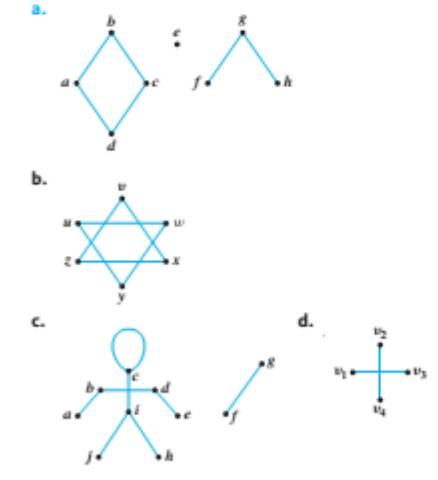To determine

(a)

Find the number of connected components for the graph below.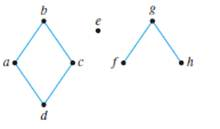Explanation

Given information:

Calculation:

In A connected component is a part of the graph where every pair of elements in this part is connected by a walk.

Each connected component contains all vertices that are connected by some walk.

We then note that the connected components are:

a, b, c and d are all connected by some walk, but not connected to any other vertices, thus {a,b,c,d}

• is a connected component.

e is not connected to any other vertices by a walk, thus {e} is a connected component

To determine

(b)

Find the number of connected components for the graph below.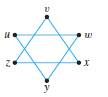To determine

(c)

Find the number of connected components for the graph below.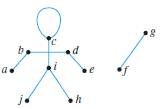To determine

(d)

Find the number of connected components for the graph below.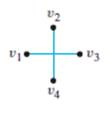### Still sussing out bartleby?

Check out a sample textbook solution.

See a sample solution

#### The Solution to Your Study Problems

Bartleby provides explanations to thousands of textbook problems written by our experts, many with advanced degrees!

Get Started